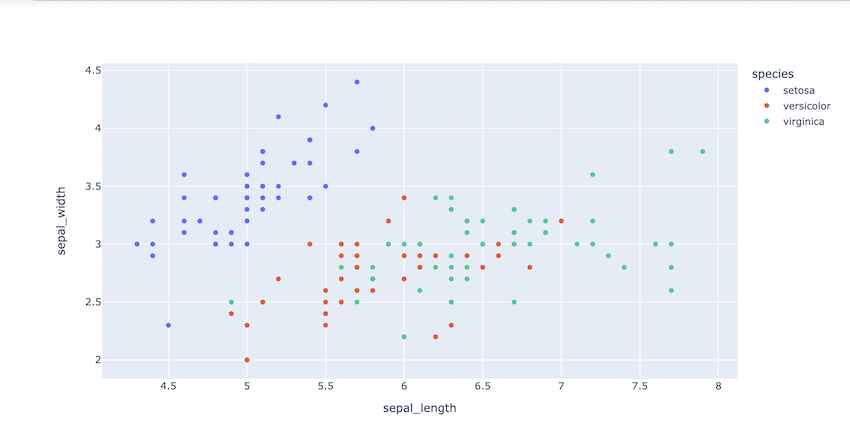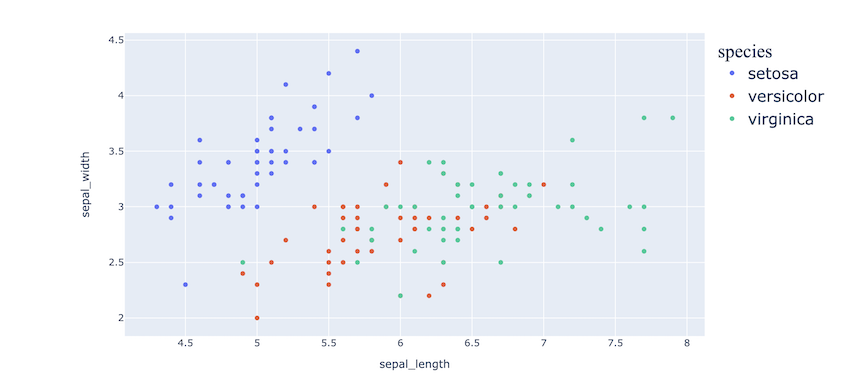# Python – Change legend size in Plotly chart

• Last Updated : 23 Jun, 2022

The data on the graph’s Y-axis, also known as the graph series, is reflected in the legend of the graph. This is the information that comes from the columns of the relevant grid report, and it usually consists of metrics. A graph legend is usually displayed as a box on the right or left side of your graph.

Plotly’s update_layout() function is used to change legend size in the plotly chart.

Syntax:

`update_layout(dict1=None, overwrite=False, **kwargs)`

The values in the input dict / keyword arguments are used to iteratively alter the parts of the original layout.

Parameters:

• dict1 (dict) – To be updated is a dictionary of properties.
• overwrite (bool) – If True, existing properties will be overwritten. If False, recursively apply updates to existing properties, retaining properties that are not specified in the update operation.
• kwargs – To be updated is a keyword/value pair of properties.

Example: Before editing size and font

Packages and CSV are imported. A plotly scatter plot is created using the px.scatter() method, X, Y, and color arguments are given. The below code is for a simple scatter plot without formatting the legend size.

## Python3

 `# import packages` `import` `plotly.express as px` `import` `pandas as pd`   `# importing csv file` `df ``=` `pd.read_csv(``"iris.csv"``)`   `# scatter plot using plotly` `fig ``=` `px.scatter(df, x``=``"sepal_length"``, y``=``"sepal_width"``, ` `                 ``color``=``"species"``)`   `fig.show()`

Output:After editing size and font:

Packages and CSV are imported. a plotly scatter plot is created using the px.scatter() method, X, Y, and color arguments are given. The below code is for creating a modified scatter plot where we use the update_layout() method to give extra parameters to our legend and change the font family and font size.

## Python3

 `# import packages` `import` `plotly.express as px` `import` `pandas as pd`   `# importing csv file` `df ``=` `pd.read_csv(``"iris.csv"``)`   `# scatter plot using plotly` `fig ``=` `px.scatter(df, x``=``"sepal_length"``, y``=``"sepal_width"``, color``=``"species"``)`   `# update_layout method used to modify change and size` `fig.update_layout(legend``=``dict``(title_font_family``=``"Times New Roman"``,` `                              ``font``=``dict``(size``=` `20``)` `))`   `fig.show()`

Output:My Personal Notes arrow_drop_up
Recommended Articles
Page :